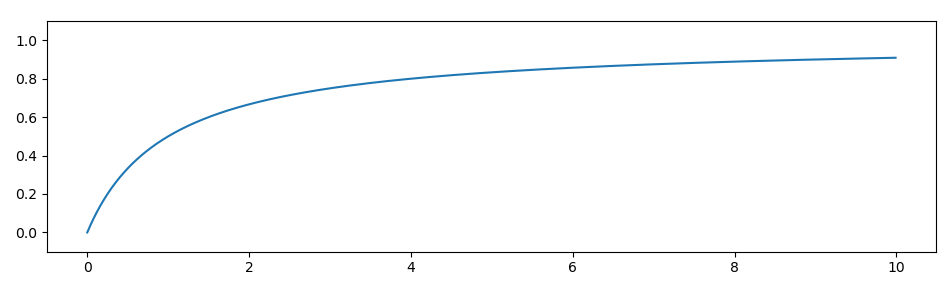시간 제한메모리 제한제출정답맞힌 사람정답 비율
2 초 (추가 시간 없음) 1024 MB83291940.426%

## 문제Today, your probability theory class covered probabilistic events that occur with high probability. As you are now explaining to your friend, this means that as some parameter $n$ goes to infinity, the probability of this event goes to $1$.

An example of an event that occurs with high probability is the following: given a list of $n$ independent uniform random integers between $1$ and $2\cdot 10^9$, there are two elements such that one divides the other.

Your friend does not believe this, as he can easily come up with many examples where no number divides any other number: Just take any subset of $\{10^9+1, 10^{9}+2, \dots, 2\cdot 10^9\}$ and none of these will divide any other!

To convince your friend, you will show him that you can find two elements that divide each other for exactly $100$ instances of the problem. You are confident this will succeed, since the probability of failure is in fact less than $10^{-25}$ per instance when $n=5\cdot 10^5$.

## 입력

The input consists of:

• One line with an integer $n$, the number of integers in the list.
• One line containing $n$ independent random integers $a_1, \ldots, a_n$, drawn uniformly from $\{1, 2, \ldots, 2\cdot 10^9\}$.

Your submission will be run on exactly $100$ test cases, all of which will have $n=5\cdot 10^5$. The samples are smaller and for illustration only.

Each of your submissions will be run on new random test cases.

## 출력

Output two distinct integers $i$ and $j$, indicating that $a_{i}$ divides $a_{j}$.

## 예제 입력 1

10
4 9 8 10 5 28 3 62 9 17


## 예제 출력 1

5 4


## 예제 입력 2

10
7 48 17 43 97 26 85 10 26 9


## 예제 출력 2

3 7


## 출처• 문제를 만든 사람: Ragnar Groot Koerkamp

## 채점 및 기타 정보

• 예제는 채점하지 않는다.